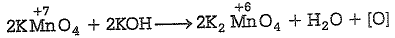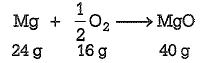# NEET Chemistry Questions Solved

How many grams of concentrated nitric acid solution should be used to prepare 250 mL of 2.0 M HNO3? The concentrated acid is 70% HNO3.

(a) 45.0 g conc. HNO3

(b) 90.0 g conc. HNO3

(c) 70.0 g conc. HNO3

(d) 54.0 g conc. HNO3

(a) Given, molarity of solution=2

Volume of solution = 250mL=250/1000=1/4L

Molar mass of HNO3 = 1+14+3x16=63g mol-1

Molarity =

Weight of  HNO3 = molarity x molecular massx volume(L) = 2x63x1/4g=31.5g

It is the weight of 100%  HNO3

But the given acid is 70% HNO3

Its weight = 31.5x100/70g=45g

Difficulty Level:

• 47%
• 27%
• 19%
• 9%

Mole fraction of the solute in a 1.00 molal aqueous solution is

(a) 0.0177

(b) 0.0344

(c) 1.7700

(d) 0.1770

(a) 1.00 molal aqueous solution = 1.0 mole in 1000g water

nsolute = 1; Wsolvent =1000g

nsolvent = 1000/18 = 55.56

Xsolute$\frac{{n}_{solute}}{{n}_{solute}+{n}_{solvent}}$

Xsolute = 1/1+55.56 = 0.0177

Difficulty Level:

• 51%
• 24%
• 16%
• 11%

6.02x1020 molecules of urea are present in 100mL of its solution. The concentration of solution is

(a) 0.02M

(b) 0.01M

(c) 0.001M

(d) 0.1M

(b) Given, number of molecules of urea= 6.02x1020

Number of moles = 6.02x1020 /NA

=6.02x1020 /6.02x1023 =1x10-3 mol

Volume of the solution = 100mL = 100/1000L =0.1L

Concentration of urea solution (in mol L-1)

=1x10-3 /0.1 mol L-1

=1x10-2 mol L-1

=0.01 mol L-1

Difficulty Level:

• 14%
• 59%
• 18%
• 11%

Which has maximum number of molecules?

(a) 7g N

(b) 2g H

(c) 16g NO

(d) 16g O

(b) In 7g nitrogen, number of  molecules = $\frac{7.0}{28}$mol = 0.25 x NA molecules

where, NA = Avogadro number = 6.023 x 1023

In 2g of H2= 2/2 mol =1x NA molecules

In 16 g of  NO2= 16/46 mol = 0.348 x NA molecules

In 16 g of O2 = 16/32 mol = 0.5 x NA molecules

Hence, maximum number of molecules are present in 2g of H2.

Difficulty Level:

• 7%
• 61%
• 25%
• 9%

The number of moles of KMnO4 reduced by one mole of KI in alkaline medium is

(a) one fifth

(b) five

(c) one

(d) two

(d) In alkaline medium,KMnO4 is reduced to K2MnO4Kl + H2$\to$KOH + HI

Hence, two mole of KMnO4 is reduced by two mole of  KI.

Difficulty Level:

• 26%
• 26%
• 31%
• 19%

The number of moles of oxygen in 1L of air containing 21% oxygen by volume, under standard conditions, is

(a) 0.0093 mole

(b) 2.10 moles

(c) 0.186 mole

(d) 0.21 mole

(a) Volume oxygen in 1L of air = 21/100x1000 = 210mL

22400 mL volume at STP is occupied by oxygen = 1mole

Therefore, number of moles occupied by 210mL = 210/22400 = 0.0093mol

Difficulty Level:

• 50%
• 20%
• 17%
• 16%

What volume of  oxygen gas (O2) measured at $0°\mathrm{C}$ and 1 atm, is needed to burn completely 1L of  propane gas (C3H8) measured under the same conditions?

(a) 7L

(b) 6L

(c) 5L

(d) 10L

(c) C3H8 + 5O2  $\to$3CO2+ 4H2O

22.4L   5x22.4L

For the combustion of 22.4L propane, oxygen required = 5 x 22.4L

For  the combustion of 1L of propane oxygen required = 5x22.4/22.4L

=5L

Difficulty Level:

• 13%
• 20%
• 60%
• 9%

1 g of magnesium is burnt with 0.56g of oxygen in a closed vessel. Which reactant is left in excess and how much?

(At. weight of Mg = 24, O=16)

(a) Mg, 0.16g

(b) O2, 0.16g

(c) Mg, 0.44g

(d) O2, 0.28g

(a) The balanced chemical equation isFrom the above equation, it is clear that, 24g of Mg reacts with 16g of O2 .

Thus,1.0 g of Mg reacts with 16/24g of O2  = 0.67g of O2

But only 0.56g of O2 is available which is less than 0.67g. Thus,O2 is the limiting reagent. Further, 16g of O2 reacts with 24g of Mg.

0.56g of O2 will react with Mg = 24/16x0.56 = 0.84g

Amount of Mg left unreacted = (1.0-0.84)g Mg = 0.16g of Mg

Difficulty Level:

• 45%
• 22%
• 27%
• 8%

Percentage of Se in peroxidase anhydrase enzyme is 0.5% by weight (at. weight = 78.4), then minimum molecular weight of peroxidase anhydrase enzyme is

(a) 1.568 x 10

(b) 15.68

(c) 2.168 x 10

(d) 1.568 x 10

(d) Suppose the molecular weight of enzyme =  x

0.5% by weight means in 100g of enzyme weight of Se=0.5g

In xg of enzyme weight of Se = $\frac{.5}{100}×x\phantom{\rule{0ex}{0ex}}$

Hence, 78.4 =

x = 15680 = 1.568x104

Difficulty Level:

• 29%
• 24%
• 14%
• 36%

Number of moles of Mn${\mathrm{O}}_{4}^{-}$ required to oxidise one mole of ferrous oxalate completely in acidic medium will be

(a) 0.6 mole

(b) 0.4 mole

(c) 7.5 moles

(d) 0.2 mole

(1) 0.6

Difficulty Level:

• 35%
• 32%
• 19%
• 16%